Processing ......FreeComputerBooks.com Links to Free Computer, Mathematics, Technical Books all over the World

Numerical Computing with MATLAB, Electronic (Web) Edition
🌠 Top Free Mathematics Books - 100% Free or Open Source!
• Title Numerical Computing with MATLAB, Electronic (Web) Edition
• Author(s) Cleve B. Moler
• Publisher: Priniting: SIAM (July 25, 2008); eBook (Updated on September 16, 2013)
• License: Reproduction of single copies of this Web edition is permitted for individual use.
• Paperback 348 pages
• Language: English
• ISBN-10: 0898716608
• ISBN-13: 978-0898716603Book Description

Numerical Computing with MATLAB is a lively textbook for an introductory course in numerical methods, MATLAB, and technical computing. The emphasis is on the informed use of mathematical software; in particular, the presentation helps readers learn enough about the mathematical functions in MATLAB to use them correctly, appreciate their limitations, and modify them appropriately.

• The book makes extensive use of computer graphics, including interactive graphical expositions of numerical algorithms. It provides more than 70 M-files, which can be downloaded from the Web. Many of the more than 200 exercises involve modifying and extending these programs.
• The topics covered include an introduction to MATLAB; linear equations; interpolation; zeros and roots; least squares; quadrature; ordinary differential equations; Fourier analysis; random numbers; eigenvalues and singular values; and partial differential equations. Motivating applications include modern problems from cryptography, touch-tone dialing, Google page-ranking, atmospheric science, and image processing, as well as classical problems from physics and engineering.

This book is designed for a one-quarter or one-semester course primarily for students in science and engineering, at the advanced undergraduate or beginning graduate level. Prerequisites are calculus, some familiarity with ordinary differential equations and matrices, and some computer programming

This Electronic (Web) Edition includes changes and corrections made since the original edition was published in 2004.

• Cleve B. Moler is Chairman and Chief Scientist at The MathWorks. Before abandoning academic life for the computer industry, Moler was a professor of mathematics and computer science for almost 20 years at the University of Michigan, Stanford University, and the University of New Mexico. He then spent five years with two computer hardware manufacturers, the Intel Hypercube organization and Ardent Computers. His professional interests center on numerical analysis and scientific computing. He is the original author of MATLAB, as well as one of the authors of the LINPACK and EISPACK scientific libraries for matrix computation.

•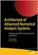Architecture of Advanced Numerical Analysis Systems

This unique open access book applies the functional OCaml programming language to numerical or computational weighted data science, engineering, and scientific applications. It's based on Owl, an OCaml-based numerical computing library.

•Tea Time Numerical Analysis: Experiences in Mathematics

This textbook was born of a desire to contribute a viable, free, introductory Numerical Analysis textbook. The ultimate goal of this book is to be a complete, one-semester, single-pdf, downloadable textbook designed for mathematics classes.

•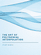The Art of Polynomial Interpolation (Stuart Murphy)

Exploring the techniques of interpolating data allows us to view the development and birth of a polynomial. This book is focused on laying a foundation for understanding and applying several common forms of Polynomial Interpolation.

•Numerical Algorithms: Computer Vision, Machine Learning, etc.

This book presents a new approach to numerical analysis for modern computer scientists, covers a wide range of topics - from numerical linear algebra to optimization and differential equations - focusing on real-world motivation and unifying themes.

•Numerical Methods and MATLAB Programming for Engineers

It covers the fundamentals while emphasizing the most essential numerical methods. Readers will enhance their programming skills using MATLAB to implement algorithms, discover how to use MATLAB to solve problems in science and engineering.

•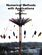Numerical Methods with Applications, 2nd Edition (Autar K Kaw)

This book entitled Numerical Methods with Applications is written primarily for engineering undergraduates taking a course in Numerical Methods. It offers a unique treatise to numerical methods which is based on a holistic approach and short chapters.

•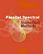Parallel Spectral Numerical Methods (Gong Chen, et al)

This book teaches the principals of Fourier spectral methods, their utility in solving partial differential equation and how to implement them in code. The programs will use Matlab and Fortran. A Python implementation of some of the Matlab programs is also provided.

•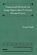Numerical Methods for Large Eigenvalue Problems (Yousef Saad)

This book is intended for researchers in applied mathematics and scientific computing as well as for practitioners interested in understanding the theory of numerical methods used for eigenvalue problems.

•First Semester in Numerical Analysis with Julia (Giray Okten)

This book presents the theory and methods, together with the implementation of the algorithms using the Julia programming language. The book covers computer arithmetic, root-finding, numerical quadrature and differentiation, and approximation theory.

•Numerical Analysis - Theory and Application (Jan Awrejcewicz)

This book is a book focused on introducing theoretical approaches of numerical analysis as well as applications of various numerical methods to either study or solving numerous theoretical and engineering problems.

•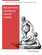High Performance Computing and Numerical Modelling

This textbook provides a step-by-step approach to numerical methods in engineering modelling. It provides a consistent treatment of the topic, from the ground up, to reinforce for students that numerical methods are a set of mathematical modelling tools.

•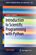Introduction to Scientific Programming with Python

This book offers an initial introduction to programming for scientific and computational applications using the Python programming language. The presentation style is compact and example-based, assuming little or no prior experience in programming.

•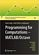Programming for Computations - MATLAB/Octave (Svein Linge)

This book presents computer programming as a key method for solving mathematical problems using MATLAB and Octave. It is intended for novice programmers. Each treated concept is illustrated and explained in detail by means of working examples.

Book Categories
 :All CategoriesRecent BooksMiscellaneous BooksComputer LanguagesComputer ScienceData Science/DatabasesElectrical EngineeringJava and Java EE (J2EE)Linux and UnixMathematicsMicrosoft and .NETMobile ComputingNetworking and CommunicationsSoftware EngineeringSpecial TopicsWeb Programming
Other Categories